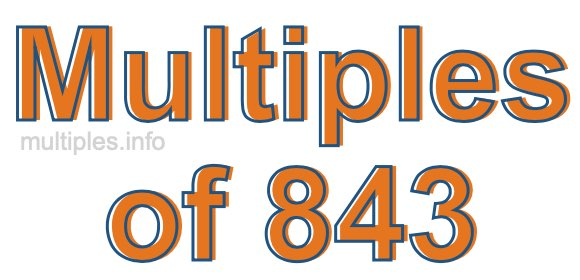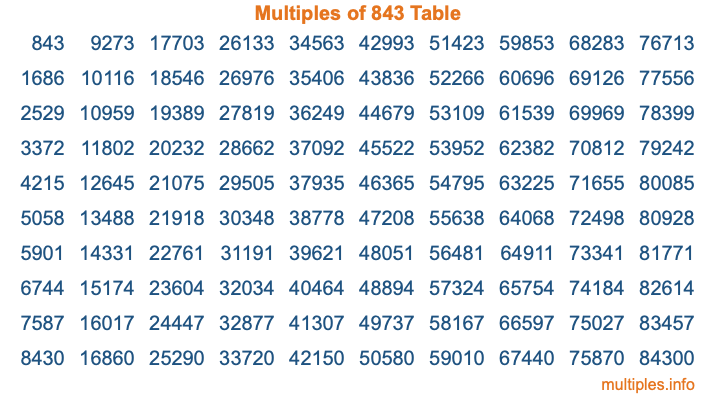Multiples of 843Welcome to the Multiples of 843 page. Here we will first teach you everything you will ever need to know about the multiples of 843, and then give you a study guide summary of everything we taught you to make sure you remember it all. Use this page to look up facts and learn information about the multiples of 843. This page will make you a multiples of eight hundred forty-three expert!

Definition of Multiples of 843
Multiples of 843 are all the numbers that when divided by 843 equal an integer. Each of the multiples of 843 are called a multiple. A multiple of 843 is created by multiplying 843 by an integer.

Therefore, to create a list of multiples of 843, you start with 1 multiplied by 843, then 2 multiplied by 843, then 3 multiplied by 843, and so on for as long as you want. Thus, the list of the first five multiples of 843 is 843, 1686, 2529, 3372, and 4215. To see a larger list of multiples of 843, see the printable image of Multiples of 843 further down on this page. We also have a category where you can choose any nth multiple of 843.

Multiples of 843 Checker
The Multiples of 843 Checker below checks to see if any number of your choice is a multiple of 843. In other words, it checks to see if there is any number (integer) that when multiplied by 843 will equal your number. To do that, we divide your number by 843. If the the quotient is an integer, then your number is a multiple of 843.

Is  a multiple of 843?

Least Common Multiple of 843 and ...
A Least Common Multiple (LCM) is the lowest multiple that two or more numbers have in common. This is also called the smallest common multiple or lowest common multiple and is useful to know when you are adding our subtracting fractions. Enter one or more numbers below (843 is already entered) to find the LCM.

Check out our LCM Calculator if you need more details about the Least Common Multiple or if you need the LCM for different numbers for adding and subtraction fractions.

nth Multiple of 843
As we stated above, 843 is the first multiple of 843, 1686 is the second multiple of 843, 2529 is the third multiple of 843, and so on. Enter a number below to find the nth multiple of 843.

th multiple of 843

Multiples of 843 vs Factors of 843
843 is a multiple of 843 and a factor of 843, but that is where the similarities end. All postive multiples of 843 are 843 or greater than 843. All positive factors of 843 are 843 or less than 843.

Below is the beginning list of multiples of 843 and the factors of 843 so you can compare:

Multiples of 843: 843, 1686, 2529, 3372, 4215, etc.

Factors of 843: 1, 3, 281, 843

As you can see, the multiples of 843 are all the numbers that you can divide by 843 to get a whole number. The factors of 843, on the other hand, are all the whole numbers that you can multiply by another whole number to get 843.

It's also interesting to note that if a number (x) is a factor of 843, then 843 will also be a multiple of that number (x).

Multiples of 843 vs Divisors of 843
The divisors of 843 are all the integers that 843 can be divided by evenly. Below is a list of the divisors of 843.

Divisors of 843: 1, 3, 281, 843

The interesting thing to note here is that if you take any multiple of 843 and divide it by a divisor of 843, you will see that the quotient is an integer.

Multiples of 843 Table
Below is an image of the first 100 multiples of 843 in a table. The table is in chronological order, column by column. The first column has the first ten multiples of 843, the second column has the next ten multiples of 843, and so on.The Multiples of 843 Table is also referred to as the 843 Times Table or Times Table of 843. You are welcome to print out our table for your studies.

Negative Multiples of 843
Although not often discussed or needed in math, it is worth mentioning that you can make a list of negative multiples of 843 by multiplying 843 by -1, then by -2, then by -3, and so on, to get the following list of negative multiples of 843:

-843, -1686, -2529, -3372, -4215, etc.

Multiples of 843 Summary
Below is a summary of important Multiples of 843 facts that we have discussed on this page. To retain the knowledge on this page, we recommend that you read through the summary and explain to yourself or a study partner why they hold true.

There are an infinite number of multiples of 843.

A multiple of 843 divided by 843 will equal a whole number.

843 divided by a factor of 843 equals a divisor of 843.

The nth multiple of 843 is n times 843.

The largest factor of 843 is equal to the first positive multiple of 843.

843 is a multiple of every factor of 843.

843 is a multiple of 843.

A multiple of 843 divided by a divisor of 843 equals an integer.

843 divided by a divisor of 843 equals a factor of 843.

Any integer times 843 will equal a multiple of 843.

Multiples of a Number
Here you can get the multiples of another number, all with the same attention to detail as we did for multiples of 843 on this page.

Multiples of
Multiples of 844
Did you find our page about multiples of eight hundred forty-three educational? Do you want more knowledge? Check out the multiples of the next number on our list!